Math resources Algebra Inequalities

Greater than sign

# Greater than sign

Here you will learn about the greater than sign, including the symbol used to represent it and how to compare numbers and fractions using the greater than sign.

Students will first learn about the greater than sign as part of the number and operations in base 10 and number and operations – fractions in elementary school.

## What is the greater than sign?

The greater than sign is a mathematical symbol used to compare numbers and expressions.

It is one of the symbols used for inequalities along with the less than symbol, <.

When stacking identical blocks, the height of 3 blocks is greater than the height of 1 block. The lines joining the stacks give the shape and direction of the inequality symbols.

The greater than sign is,

The greater than sign is also known as the more than sign. The wide end of the symbol always faces the bigger number or expression – the symbol looks open towards the bigger number and ‘points’ at the smaller value like an arrow.

For example,

This is read as ‘10 is greater than 6’.

The greater than sign is used to compare;

Whole numbers
15 > 10
is greater than 10’.
Decimals
2.3 > 1.8
This is read as ‘2.3 is
greater than 1.8’.
Fractions
\cfrac{1}{2} \, >\cfrac{1}{3}
This is read as ‘one half is
greater than one third’.

### What is the greater than sign?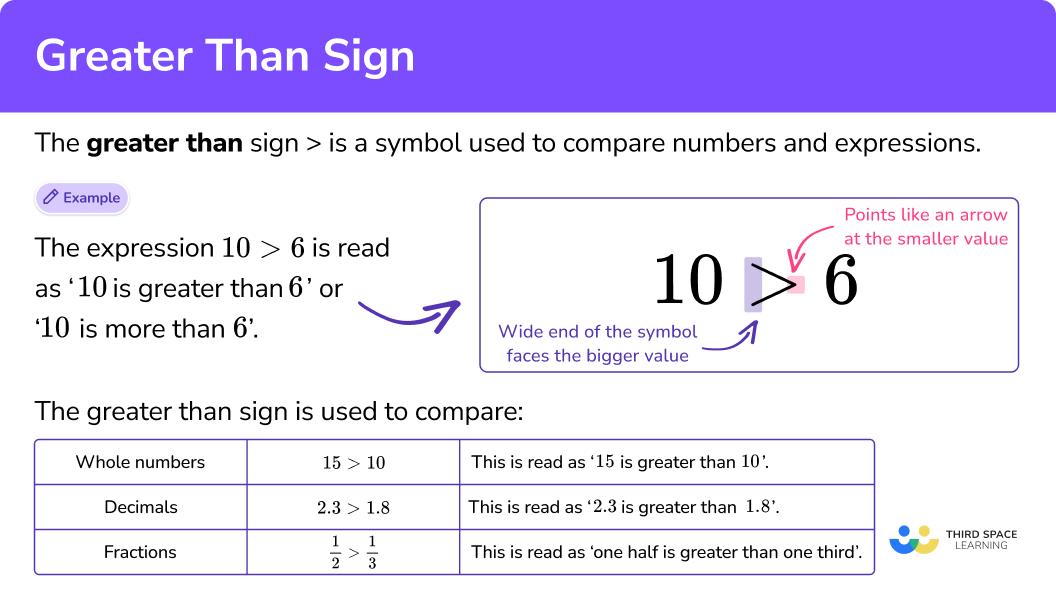## Common Core State Standards

• Grade 1: Number and Operations in Base Ten (1.NBT.B.3)
Compare two two-digit numbers based on meanings of the tens and ones digits, recording the results of comparisons with the symbols >, \, =, and <.

• Grade 2: Number and Operations in Base Ten (2.NBT.A.4)
Compare two three-digit numbers based on meanings of the hundreds, tens, and ones digits, using >, \, =, and < symbols to record the results of comparisons.

• Grade 3: Number and Operations – Fractions (3.NF.A.3d)
Compare two fractions with the same numerator or the same denominator by reasoning about their size. Recognize that comparisons are valid only when the two fractions refer to the same whole. Record the results of comparisons with the symbols >, \, =, or <, and justify the conclusions, for example, by using a visual fraction model.

• Grade 4: Number and Operations in Base Ten (4.NBT.A.2)
Read and write multi-digit whole numbers using base-ten numerals, number names, and expanded form. Compare two multi-digit numbers based on meanings of the digits in each place, using >, \, =, and < symbols to record the results of comparisons.

• Grade 4: Number and Operations – Fractions (4.NF.A.2)
Compare two fractions with different numerators and different denominators, e.g., by creating common denominators or numerators, or by comparing to a benchmark fraction such as \frac{1}{2}. Recognize that comparisons are valid only when the two fractions refer to the same whole. Record the results of comparisons with symbols > , \, =, or <, and justify the conclusions, for example, by using a visual fraction model.

• Grade 5: Number and Operations in Base Ten (5.NBT.3b)
Compare two decimals to thousandths based on meanings of the digits in each place, using >, \, =, and < symbols to record the results of comparisons.

## How to compare values using the greater than sign

In order to compare values using the greater than sign:

1. Write the given numbers into a place value chart.
2. Starting with the greatest place value, compare the digits of the number, until you find digits that are different.
3. Write the values with the correct symbol, or place the numbers on the correct sides of a given symbol.

## Greater than sign examples

### Example 1: comparing two-digit numbers

Write the correct sign, > or <, in the box.

1. Write the given numbers into a place value chart.

2Starting with the greatest place value, compare the digits of the number, until you find digits that are different.

Looking at the place value chart, start comparing the greatest place value, the tens place.

32 has 3 tens and 17 has 1 ten. You know that 3 tens is greater than 1 ten, therefore, 32 is the greater number.

3Write the values with the correct symbol, or place the numbers on the correct sides of a given symbol.

32 is greater than 17.

Place the wide end of the symbol towards the 32, with the point facing the smaller number, 17.

### Example 2: comparing three-digit numbers

Write the correct sign, > or <, in the box.

Write the given numbers into a place value chart.

Starting with the greatest place value, compare the digits of the number, until you find digits that are different.

Write the values with the correct symbol, or place the numbers on the correct sides of a given symbol.

### Example 3: comparing multi-digit numbers

Write the correct sign, > or <, in the box.

Write the given numbers into a place value chart.

Starting with the greatest place value, compare the digits of the number, until you find digits that are different.

Write the values with the correct symbol, or place the numbers on the correct sides of a given symbol.

### Example 4: comparing decimals

Write the correct sign, > or <, in the box.

Write the given numbers into a place value chart.

Starting with the greatest place value, compare the digits of the number, until you find digits that are different.

Write the values with the correct symbol, or place the numbers on the correct sides of a given symbol.

## How to compare fractions using the greater than sign

In order to compare fractions using the greater than sign:

1. See if the fractions have equal numerators or denominators.
2. Use equivalent fractions to find a common denominator if needed.
3. Compare the fractions and write the answer using the original fractions and correct comparison symbol.

### Example 5: comparing fractions with same numerator

Which is larger, \, \cfrac{4}{5} \, or \, \cfrac{4}{8} \, ? Write your answer using the greater than comparison symbol.

See if the fractions have equal numerators or denominators.

Use equivalent fractions to find a common denominator if needed.

Compare the fractions and write the answer using the original fractions and correct comparison symbol.

### Example 6: comparing fractions using common denominator

Which is larger, \, \cfrac{5}{6} \, or \, \cfrac{7}{12} \, ? Write your answer using the greater than comparison symbol.

See if the fractions have equal numerators or denominators.

Use equivalent fractions to find a common denominator if needed.

Compare the fractions and write the answer using the original fractions and correct comparison symbol.

### Example 7: comparing fractions using common denominator

Which is larger, \, \cfrac{3}{5} \, or \, \cfrac{2}{7} \, ? Write your answer using the greater than comparison symbol.

See if the fractions have equal numerators or denominators.

Use equivalent fractions to find a common denominator if needed.

Compare the fractions and write the answer using the original fractions and correct comparison symbol.

### Teaching tips for greater than sign

• When students are first learning to compare numbers, it’s important to allow them to investigate with a hands-on approach. For students in higher grade levels who are continuing to struggle, manipulatives are a great way to reinforce meaning. There will be time for worksheets as practice after students have a better understanding of what it means to compare numbers.

• It is important for students to understand what each symbol means when comparing numbers. These symbols can be displayed in the classroom on an anchor chart or in interactive notebooks while students get familiar with the meanings.

• Although drawing an alligator’s mouth may be amusing for younger primary school kids, they often focus solely on the higher number rather than the complete comparison. Students should be reminded to utilize the math symbols as intended and to emphasize their meanings each time they are used.

### Easy mistakes to make

• Confusing the greater than and less than symbols
The biggest mistake is writing the symbols the wrong way round. Remember, the sign should point at the smallest number like an arrow.
For example, 14 \, > \, 7, \, ‘14 is greater than 7’, or 7 \, < \, 14, \, ‘7 is less than 14’.

• Trying to compare fractions without common numerator or denominator
When comparing fractions, the fractions should have either a common numerator or denominator. You may have to convert them into equivalent fractions with common denominators, so you can compare the numerators.

This greater than sign topic guide is part of our series on inequalities. You may find it helpful to start with the main inequalities topic guide for a summary of what to expect or use the step-by-step guides below for further detail on individual topics. Other topic guides in this series include:

### Practice greater than sign questions

1. Which is larger, 57 or 75? Write your answer using the greater than comparison symbol.

57 > 7557 < 7575 < 5775 > 57Write the numbers you are comparing into a place value chart.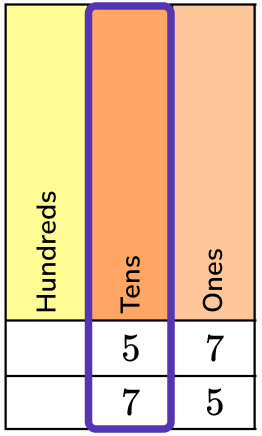Start with the greatest place value chart, the tens, and compare the digits.

57 has 5 tens and 75 has 7 tens.

7 tens is greater than 5 tens.

75 is greater than 57.

75 > 57.

2. Which is larger, 423 or 432? Write your answer using the greater than comparison symbol.

432 < 423432 > 423423 > 432423 < 432Write the numbers you are comparing into a place value chart.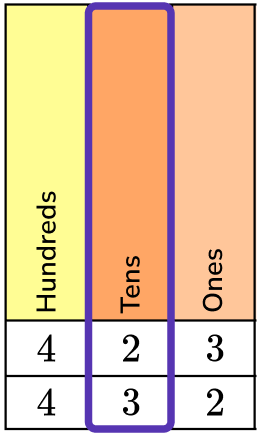The digits in the hundreds place are the same, so you would move to the tens place to compare.

423 has 2 tens and 432 has 3 tens.

3 tens is greater than 2 tens.

432 is greater than 423.

432 > 423.

3. Which is larger, 34,568 or 34,768? Write your answer using the greater than comparison symbol.

34,768 > 34,56834,768 < 34,56834,568 > 34,76834,568 < 34,768Write the numbers you are comparing into a place value chart.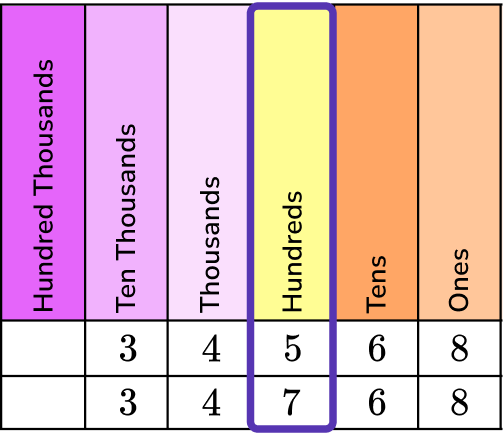The digits in the ten thousands place and thousands place are the same, so you would move to the hundreds place to compare.

34,568 has 5 hundreds and 34,768 has 7 hundreds.

7 hundreds is greater than 5 hundreds.

34,768 is greater than 34,568.

34,768 > 34,568.

4. Which is larger, 6.09 or 6.90? Write your answer using the greater than comparison symbol.

6.90 > 6.096.90 < 6.096.09 > 6.906.09 < 6.90Write the numbers you are comparing into a place value chart.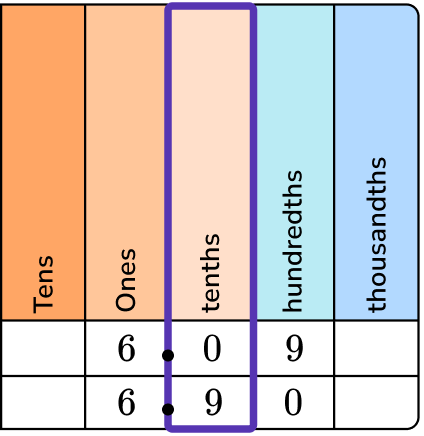The digits in the ones place are the same, so you would move to the tenths place to compare.

6.09 has 0 tenths and 6.90 has 9 tenths.
9 tenths is greater than 0 tenths.

6.90 is greater than 6.09.

6.90 > 6.09.

5. Which is larger, \, \cfrac{4}{7} \, or \, \cfrac{4}{9} \, ? Write your answer using the greater than comparison symbol.

\cfrac{4}{9} \, > \, \cfrac{4}{7}\cfrac{4}{9} \, < \, \cfrac{4}{7}\cfrac{4}{7} \, > \, \cfrac{4}{9}\cfrac{4}{7} \, < \, \cfrac{4}{9}The fractions have equal numerators, so you do not need to make equivalent fractions.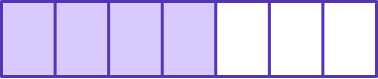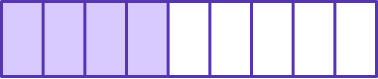Sevenths are larger, so 4 sevenths will be greater than 4 ninths.

Therefore, \, \cfrac{4}{7} \, is greater than \, \cfrac{4}{9} \, .

\cfrac{4}{7} \, > \, \cfrac{4}{9} \, .

6. Which is larger, \, \cfrac{3}{4} \, or \, \cfrac{7}{8} \, ? Write your answer using the greater than comparison symbol.

\cfrac{3}{4} \, < \, \cfrac{7}{8}\cfrac{7}{8} \, > \, \cfrac{3}{4}\cfrac{3}{4} \, > \, \cfrac{7}{8}\cfrac{7}{8} \, < \, \cfrac{3}{4}The fractions do not have the same numerators (top numbers) or denominators (bottom numbers).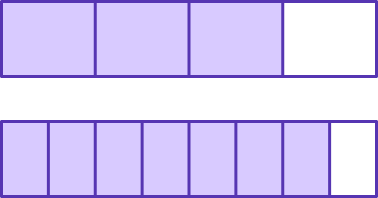To create a common denominator, double the fraction \cfrac{3}{4} to make the common denominator of 8.

\cfrac{3}{4}=\cfrac{3 \, \times \, 2}{4 \, \times \, 2}=\cfrac{6}{8}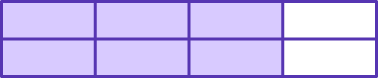Compare the fractions with common denominators \, \cfrac{6}{8} \, and \, \cfrac{7}{8} \, to determine which is larger.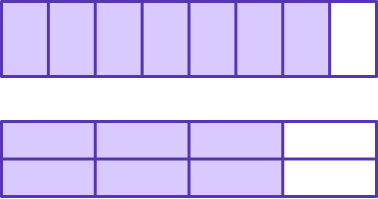\cfrac{6}{8} \, has 8 parts shaded in and \, \cfrac{7}{8} \, has 7 parts shaded in.

Since the parts are the same size, \, \cfrac{7}{8} \, is larger.

\cfrac{7}{8} \, is larger than \, \cfrac{3}{4} \, .

\cfrac{7}{8} \, > \, \cfrac{3}{4} \, .

## Greater than sign FAQs

What’s the difference between the greater than and less than sign?

When using the greater than sign, the first number will be the largest number followed by the second number being the smaller of the two numbers. The two numbers will be separated by the greater than sign, >.

What other inequality symbols are there besides the greater than sign?

When comparing numbers, you will also use the less than sign, <, the equal sign, =, the less than or equal to sign, ≤, and the greater than or equal to sign, ≥ .

## Still stuck?

At Third Space Learning, we specialize in helping teachers and school leaders to provide personalized math support for more of their students through high-quality, online one-on-one math tutoring delivered by subject experts.

Each week, our tutors support thousands of students who are at risk of not meeting their grade-level expectations, and help accelerate their progress and boost their confidence.